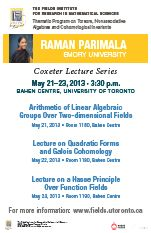# THEMATIC PROGRAMSMay 24, 2019THE FIELDS INSTITUTE FOR RESEARCH IN MATHEMATICAL SCIENCES
20th ANNIVERSARY YEAR

 January–June 2013 Thematic Program on Torsors, Nonassociative Algebras and Cohomological Invariants Coxeter Lecture Series, May 21-23 Raman Parimala, Emory University Lecture on Arithmetic of linear algebraic groups over two dimensional fields May 21, 2013 at 3:30 p.m. Bahen Centre, Rm 1180 (map) Lecture on Quadratic forms and Galois cohomology May 22, 2013 at 3:30 p.m. Bahen Centre, Rm 1190 (map) Lecture on A Hasse principle over function fields May 23, 2013 at 3:30 p.m. Bahen Centre, Rm 1190 (map)Lecture I: Arithmetic of linear algebraic groups over two dimensional fields (presentation slides) A conjecture of Serre (Conjecture II) states that every principal homogeneous space under a semisimple simply connected linear algebraic group, defined over a field of cohomological dimension two, has a rational point. This conjecture for totally imaginary number fields, known as the Hasse principle conjecture, is settled by Kneser-Harder-Chernousov. A major breakthrough for general fields is a theorem of Merkurjev-Suslin which settles Conjecture II for groups of inner type An. I will describe some approaches to resolving this conjecture, each yielding a positive answer to Conjecture II for special classes of groups or fields. Lecture II: Quadratic forms and Galois cohomology (presentation slides) The classical invariants of quadratic forms, the dimension, the discriminant and the Clifford invariant classify quadratic forms over totally imaginary number fields. Milnor proposed successive higher invariants for quadratic forms with values in mod 2 Galois cohomology groups, extending the classical invariants, and he conjectured that these invariants classify quadratic forms up to isomorphism. Milnor’s conjecture is a theorem due to Voevodsky, Orlov and Vishik. Using this theorem, bounds on the generation of Galois cohomology groups lead to arithmetic consequences. Among them is the finiteness of the u-invariant, equivalently, that quadratic forms in sufficiently many variables represent zero nontrivially. I will discuss some progress towards the determination of the u-invariant of function fields of curves over fields of arithmetic interest. Lecture III: A Hasse principle over function fields (presentation slides) A local-global principle for the existence of nontrivial zeros of quadratic forms over function fields, with respect to completions at discrete valuations, has interesting consequences. For function fields of curves over finite fields, it gives the classical theorem of Hasse-Minkowski. One could look for a more general Hasse principle for the existence of rational points on homogeneous spaces under connected linear algebraic groups defined over function fields of curves over a local or a global field. I will explain some positive results in this direction for certain classes of groups which include split simply connected groups over function fields of curves over local fields. There are recent examples of the failure of the Hasse principle for rational points on principal homogeneous spaces under nonrational tori defined over function fields of p-adic curves. This leads to the question whether the Hasse principle holds for all semisimple simply connected groups over these fields. Speakers in the Distinguished Lecture Series (DLS) have made outstanding contributions to their field of mathematics. The DLS consists of a series of three one-hour lectures.